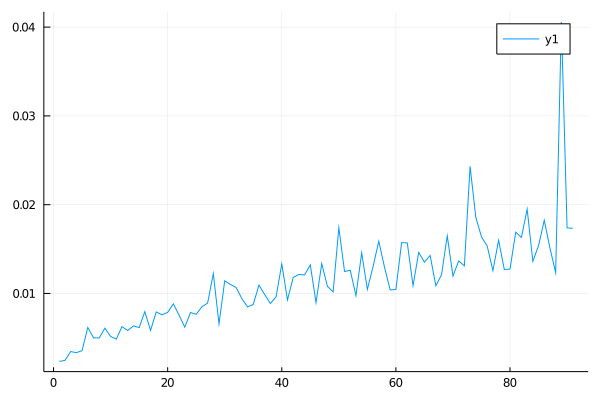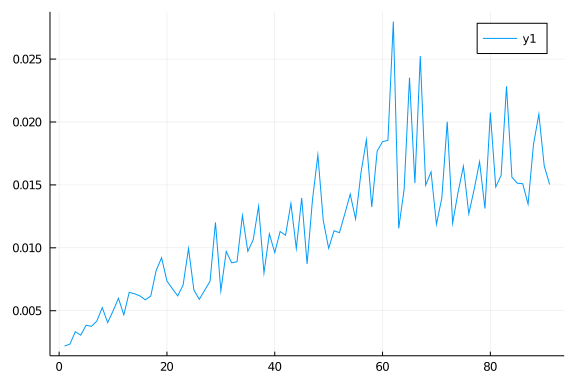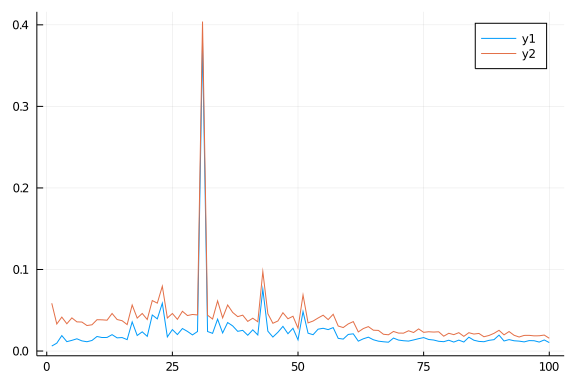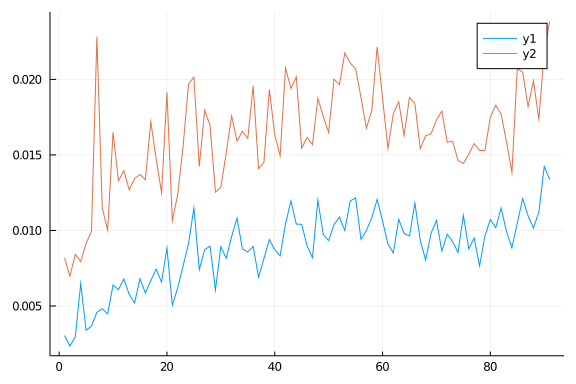# A tale of two knapsacks

A tale of two Knapsacks

# A tale of two Knapsacks

By the end of this post, you will know what cover cuts are and how to implement them in optimization packages such as JuMP (Julia for Mathematical Programming). Those familiar with the definitions and interested only in learning about the implementation details can skip to the section on branch-n-cut. We assume that the reader is familiar with branch-n-bound in integer programming. We will use only the generic callbacks in JuMP. Solver (CPLEX, Gurobi, GLPK) specific callbacks will not be used.

We begin with definitions of the two problems and integer programs for them. Subsequently, we examine the use of "cover-cuts" to solve maximum knapsack. The "cover-cuts" are generated by solving a minimum knapsack instance. This will yield a branch-n-cut algorithm for solving maximum knapsack. We will explore this relationship between the two problems in some detail and see how to use lazy constraints to implement a branch-n-cut algorithm in JuMP v0.21.1.

# Maximum Knapsack

The maximum knapsack is simply stated NP-complete "easy" problem. It's easy in the sense that massive instances can be solved in seconds in practice. An instance of a maximum knapsack is a set of items. A profit and a size are associated with each item. Given is a knapsack of limited capacity, the goal is to pick the subset of most profitable things that fit in the knapsack.

There are n items indexed from 1..n. Arrays p and s store the profits and the sizes of the items. Indicator variable $x_i$ models whether item i is in the knapsack or not. The integer program below for maximum knapsack is solved using the MIP solver in Gurobi.

$\mbox{max} \sum_{i \in [1..n]} p_i x_i$

$\sum_{i \in [1..n]} s_i x_i \le C$

$x_i \in \{0,1\} \mbox{ for all } i \in [1..n]$

Function maxks below uses the JuMP library to create and solve a mathematical model for the knapsack problem. Let's start by importing the two libraries; JuMP for domain-specific modelling language and Gurobi for an LP and MIP solver.

julia> using JuMP, Gurobi

julia> const GRB_ENV = Gurobi.Env() # reuse the Gurobi environment
Gurobi.Env(Ptr{Nothing} @0x000000000323f440, false, 0)


The generic steps are to load the solver, specify the model, and optimize the model. The function below will find an optimal solution to a maximum knapsack instance specified by the arrays of profits, sizes, and the knapsack's capacity using GLPK. Model is used to create an integer program to be solved by the Gurobi solver with options as below. JuMP macros @variable, @constraint, @objective are used to create variables, constraint and the objective function. optimize! solves the named model. The ks model created below is an integer program because the variables are of type binary (Bin). We will use the Gurobi solver with presolve, cuts, and heuristics disabled, and lazy constraints enabled so that only our cuts are used. PreCrush option has to be set to 1 to tell Gurobi that there are user cuts and not use reductions to transform constraints. We will use a single thread for Gurobi.

julia> function maxks(profits, sizes, capacity)
n = length(sizes)
ks = Model(() -> Gurobi.Optimizer(GRB_ENV))
set_optimizer_attributes(ks, "LazyConstraints" => 1,
"PreCrush" =>1, "Cuts" => 0, "Threads" => 1,
"Presolve" => 0, "Heuristics" => 0.0, "OutputFlag" => 0)
@variable(ks, x[1:n], Bin )
@constraint(ks, sum(sizes[i]* x[i] for i in 1:n ) <= capacity)
@objective(ks, Max, sum(profits[i]*x[i] for i in 1:n))
optimize!(ks)
return (objective_value(ks), [value(x[i]) for i in 1:n])
end
maxks (generic function with 1 method)


Let's see how "easy" maximum knapsack is? It is non-trivial to find instances where popular heuristics are not effective. We examine two categories of "hard" examples from the paper titled "Where are the hard knapsack problems?" The instances are the circle and the profit ceiling instances. The sizes are generated uniformly at random in the range 1:R. The profits are generated as shown below. For each n, 20 instances are generated, and the capacity of instance numbered j is j/21. It appears that for the MIP solver, profit ceiling instances are harder. You can uncomment the line profits = [2/3 * sqrt( 4*R^2 - ( sizes[k] - 2*R)^2 ) for k=1:i] in the function instance to generate profits for the circle instances and check.

julia> function instance(i)  # with i items
R = 1000 # circle & profit ceiling instances
sizes = rand(1:R, i)
# profits = [2/3 * sqrt( 4*R^2 - ( sizes[k] - 2*R)^2 ) for k=1:i]
profits = [ 3 * ceil(sizes[k]/3) for k in 1:i]
return (sizes, profits)
end
instance (generic function with 1 method)


In the plot below, the x-axis is the size of the instances (number of items) from 10 to 100 in steps of 1. The average time (sec) taken is on the y-axis. Relatively large instances can be solved in a matter of milliseconds.# Minimum Knapsack

The minimum knapsack is a dual of sorts to the maximum knapsack. The items come with sizes and profits as earlier. Also given a knapsack with minimum capacity C. The goal is to pick a subset of items with a total size of at least $C$ whose profit is minimum possible (think costs instead of profits). The integer program for minimum knapsack is below. The function minks is similar to maxks in strucutre save the inequality in the constraint and the minimization objective. There might not be any feasible solution, therefore if the IP is infeasible then we return (-1,[]).

$\mbox{min} \sum_{i \in [1..n]} p_i y_i$

$\sum_{i \in [1..n]} s_i y_i \ge C$

$y_i \in \{0,1\} \mbox{ for all } i \in [1..n]$

julia> function minks(costs, sizes, capacity)
n = length(sizes)
ks = Model(() -> Gurobi.Optimizer(GRB_ENV))
set_optimizer_attributes(ks, "LazyConstraints" => 1,
"PreCrush" =>1, "Cuts" => 0, "Threads" => 1,
"Presolve" => 0, "Heuristics" => 0.0 ,"OutputFlag" => 0)
@variable(ks, y[1:n], Bin )
@constraint(ks, sum(sizes[i]* y[i] for i in 1:n ) >= capacity)
@objective(ks, Min, sum(costs[i]*y[i] for i in 1:n))
optimize!(ks)
if (termination_status(ks) != MOI.OPTIMAL) return (-1,[]) end
return (objective_value(ks), value.(y))
end
minks (generic function with 1 method)


We solve the integer program using the Gurobi solver. In the plot below, the x-axis is the size of the instances (number of items) from 10 to 100 in steps of 1. Twenty instances are created for $n$, and the capacity is as above. The average time (in seconds) is on the y-axis.n For every $n$, twenty instances are used to determine the average.Even the "difficult" instances are easy to solve for modern MIP solvers. The running time for minimum knapsack is smaller compared to the time for maximum knapsack. What we have learned so far is that knapsacks are easy to solve in practice using branch-n-bound (integer programming) and minimum knapsack is relatively "easier" than maximum knapsack.

# Cover-Cuts

If we remove the integrality constraint, $x_i \in \{0,1\}$, and add the constraints that $0 \le x_i \le 1$ for all $i$ then the resulting program is called linear programming (LP) relaxation of the integer program. An optimal solution to the LP relaxation is referred to as an LP solution.

A set of items $S$ is said to fit in the knapsack if $\sum_{i \in S} s_i \le C$ where $C$ is the knapsack capacity. A minimal cover $S$ is a subset of items that do not fit in the knapsack, but any proper subset of the cover fits in the knapsack. From every minimal cover $S$, the knapsack can contain at most $|S|-1$ items. This constraint is called the cover constraint and can be written as

$\sum_{i \in S} x_i \le |S|-1$

where subset of items $S$ is a cover. This equation can be rewritten as

$\sum_{i \in S} (1-x_i) \ge 1. \hspace{4cm} (*)$

Any 0/1 solution to the maximum knapsack solution has to satisfy the cover constraint above. An LP solution also satisfies the cover condition. Let the current LP solution be $x^*,$ we need to find a cover-cut, a subset of items $S$ that forms a cover but does not satisfy $(*)$.

To restate, we want a subset of items $S$ which satisfies the following two constraints.

$\sum_{i \in S} (1-x_i^*) < 1$

$\sum_{i \in S} s_i > C$

The problem of finding $S$ is again a maximum knapsack problem with a knapsack of capacity $1-\epsilon$ where item $i$ has size $(1-x_i^*)$ and profit $s_i$. Let $y_i$ be the decision variables. $S$ contains all those items for which $y_i = 1$. If there is an optimal solution to the integer program below with objective function value $> C$ then we have a cover-cut. We can now add the constraint $\sum_{i \in S} x_i \le |S| -1$ to the LP relaxation (or IP).

$\mbox{max} \sum_{i \in [1..n]} s_i y_i$

$\sum_{i \in [1..n]} y_i (1-x_i^*) \le 1 - \epsilon$

Solving the knapsack problem above gives us a cover constraint that is violated by the current LP solution $x^*$. To solve maximum knapsack, we have to solve another maximum knapsack, what do we gain? The gain, if any is realized because the new instance of knapsack is "easy" to solve.

Such an $S$ (which is a cover & violates $(*)$) can also be computed by solving a minimum knapsack instance (assuming sizes are integers)

$\mbox{min} \sum_{i \in [1..n]} (1-x_i^*) y_i$

$\sum_{i \in [1..n]} s_i y_i \ge C+1$

If the objective function value above is $< 1$ then we have a cover-cut. The function maxks_cuts below will repeatedly solve a minimum knapsack problem to find cover constraints violated by the current LP solution. These cuts are then added to the model, and the LP is resolved. The process continues until no more cuts can be added. Starting with an LP relaxation, we can increase the value of the objective function by adding more and more cuts.

Computation of cuts using both maximum as well as minimum knapsack is shown below. See the commented part in the code below. The maximum knapsack based formulation is always feasible and returns an optimal solution. The minimum Knapsack formulation may not be feasible, so we cannot simply check against the value of the optimal solution. It is essential to check whether minimum knapsack returns an optimal solution. It should also be clear from this comment that even after adding all the cover-cuts, the polytope need not be integral.

julia> function maxks_cuts(profits, sizes, capacity)
n = length(sizes)
ks = Model(() -> Gurobi.Optimizer(GRB_ENV))
set_optimizer_attributes(ks, "LazyConstraints" => 1,
"PreCrush" =>1, "Cuts" => 0, "Threads" => 1,
"Presolve" => 0, "Heuristics" => 0.0)
@variable(ks, x[1:n], Bin )
relax_integrality(ks)               # LP relaxation

@constraint(ks, sum(sizes[i]*x[i] for i in 1:n ) <= capacity)
@objective(ks, Max, sum(profits[i]*x[i] for i in 1:n))
optimize!(ks)                         # solve the LP

x1 = []
while true
x1 = [value(x[i]) for i in 1:n]
(obj, y) = minks(1 .- x1, sizes, capacity+1) # solve IP for cut
((obj < 1) && ( y != [])) || break # cut generation failed

## cut based on  max knapsack (always feasible)
## (obj, y) = maxks(sizes, 1 .- x1, 1 - 0.01)
## (obj >= capacity+1) || break
##
S = [j for j in 1:n if y[j] > 0]
@constraint(ks, sum(x[i] for i in S) <= length(S) - 1)
optimize!(ks)
end

# print(ks) $print the model. return (objective_value(ks), x1) end maxks_cuts (generic function with 1 method)  Function maxks_cuts solves the LP relaxation of maximum knapsack by repeated adding cover-cuts. The cover-cuts are computed by solving a minimum knapsack instance. An exponential number of cover-cuts might be added. # Branch-n-Cut The process of addition of cuts chips the polytope away. This process can be performed at every node in the branch and bound search tree. Optimizers such as CPLEX, Gurobi and GLPK provide hooks to automate the additions of cuts to the nodes in the search tree. These cuts are called lazy constraints and the hooks are called callbacks. Next, we will see how to use callbacks to automate the addition of lazy constraints (cuts) in JuMP. To add a hook, one needs two simple steps. The first step is to write a function, cover_cut, that will generate the cut given the current solution. The second step is to tell JuMP about the callback function using MOI.set. The function cover_cut needs the current LP solution and the instance data (sizes, capacity). However by design, cover_cut takes only one argument traditionally called cb_data (callback data), which is used by the handler and callback_value is the only function available which can be used to query the values of the variables in the model. One can use global variables to pass instance-specific data to cover_cut; another approach is to define the cover_cut function inside the solver for maximum knapsack (maxks_cut_lazy). This allows the instance data to visible inside cover_cut given the scoping rules of Julia. This is what we do below. MOI.set(ks, MOI.LazyConstraintCallback(), cover_cut) specifies that cuts are to be generated by cover_cut. Lazy constraints may remove integral points from the polytope, whereas lazy cuts do not remove any integral points. See JuMP manual for more on this distinction. julia> function maxks_cuts_lazy(profits, sizes, capacity) n = length(sizes) ks = Model(() -> Gurobi.Optimizer(GRB_ENV)) set_optimizer_attributes(ks, "LazyConstraints" => 1, "PreCrush" =>1, "Cuts" => 0, "Threads" => 1, "Presolve" => 0, "Heuristics" => 0.0 ,"OutputFlag" => 0) @variable(ks, x[1:n], Bin ) # x..x[N] Binary @constraint(ks, sum(sizes[i]*x[i] for i in 1:n ) <= capacity) @objective(ks, Max, sum(profits[i]*x[i] for i in 1:n)) function cover_cut(cb_data) x_vals = [callback_value(cb_data, x[i]) for i in 1:n] # LP solution (obj, y) = minks(1 .- x_vals, sizes, capacity+1) ((obj < 1) && ( y != [])) || return S = [j for j in 1:n if y[j] > 0] con = @build_constraint(sum(x[i] for i in S) <= length(S) - 1) MOI.submit(ks, MOI.LazyConstraint(cb_data), con) end MOI.set(ks, MOI.LazyConstraintCallback(), cover_cut) # add cut at each LP node optimize!(ks) return (objective_value(ks), value.(x), ks) end maxks_cuts_lazy (generic function with 1 method)  Next, we solve using a built-in branch and bound with no optimizations and automatics cuts disabled running on a single thread and compare it to the method which adds cover-cuts at all the nodes in the search tree. The resulting algorithm is called branch and cut. The tables below are the benchmark results [on Intel(R) Core(TM) i5-4210U CPU @ 1.70GHz] for the branch-n-cut and the standard MIP solver on an input of size$100$. The knapsack capacity is$j/101*S$where$j$varies from 1 to 100, and$S$is the total size of all the items. The x-axis is$j$, and the y-axis is the average time taken by the two methods in seconds. The average time is determined by a set of 20 randomly generated instances. The conventional wisdom says that maximum knapsack is "easy" and cover-cuts are an overkill. This is indeed what we see in the figure below. The LP relaxation without cover-cuts provides a "good enough" bound for branch-n-bound search.The figure below shows the time taken by the two solvers on inputs of size ranging from 10 to 100. The instances are generated as earlier. For every$n$, 20 instances are generated with the same sizes and profits using the function instance(n). The capacity, for instance$j$is$0.85\$ fraction of the total size of all the items. The average time taken by the two algorithms on the 20 instances is shown below. The branch and cut solver with cover-cut constraints is slower than the default branch-n-bound scheme.Note that this method of implementing branch-n-cut does not provide fine-grained control. The solver is not obligated to use the cuts posted by the code (in cover_cut). It is not clear to the author how the cuts are used when branch-n-bound is using multiple threads in Gurobi. CPLEX provides better control, and the SCIP framework provides the most control.

Thanks to Vijay Adoni & Leila Karimi for noting a typo in the minks function in an earlier version. This is a rerun.